# CSquare

•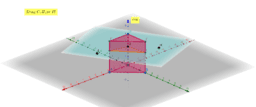### 3d

Activity

CSquare

•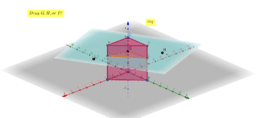### Cross Section Triangular Prism

Activity

CSquare

•### Cross Section Rectangular Prism

Activity

CSquare

•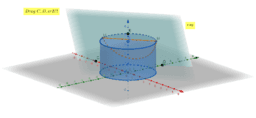### Cross Section Cylinder

Activity

CSquare

•### Cross Sections Cone

Activity

CSquare

•### Cross Section Pyramid

Activity

CSquare

•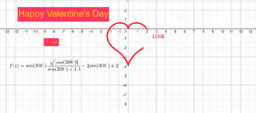### Happy Valentine's Day

Activity

CSquare

•### Graphing Linear Equations Using Slope and y-intercept (Pract

Activity

CSquare

•### Constructing a Parallel Line through a Point to a given Line

Activity

CSquare

•### Writing Equations of Parallel Lines (Practice)

Activity

CSquare

•### Factoring Trinomials with leading coeficient a =1

Activity

CSquare

•### Partitioning a Line Segment Level 1

Activity

CSquare

•### Distance Formula/Length of a Segment(Practice)

Activity

CSquare

•### Perpendicular Line and Their Slopes

Activity

CSquare

•### Writing Equations of Perpendicular Lines (Practice)

Activity

CSquare

•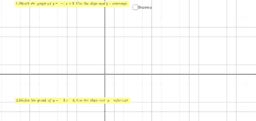### Parallel Lines and Their Slopes (Discovery)

Activity

CSquare

•### Partitioning a Line Segment Level 2

Activity

CSquare

•### Absolute Value Equations Part 2 (Practice)

Activity

CSquare

•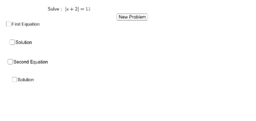### Absolute Value Equations Part 1 (Practice)

Activity

CSquare

•### Midpoint and Endpoint (Practice)

Activity

CSquare

•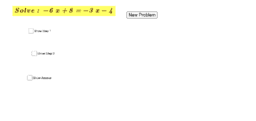### Two Steps Equations

Activity

CSquare

•### Finding the Midpoint (Practice)

Activity

CSquare

•Activity

CSquare